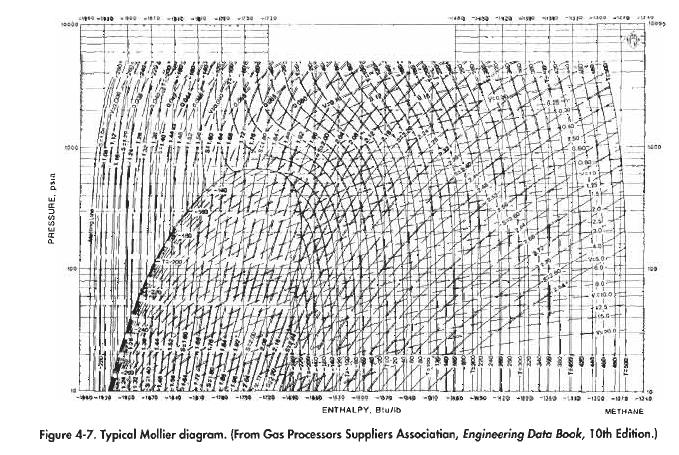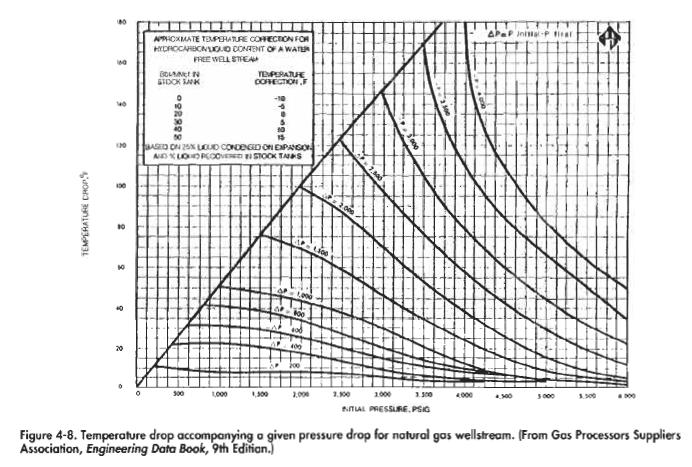﻿ Temperature Drop Due To Gas Expansion | Oil & Gas Process Engineering

Temperature Drop Due To Gas Expansion

Choking, or expansion of gas from a high pressure to a lower pressure, is generally required for control of gas flow rates. Choking is achieved by the use of a choke or a control valve. The pressure drop causes a decrease in the gas temperature, thus hydrates can form at the choke or control valve. The best way to calculate the temperature drop is to use a simulation computer program. The program will perform a flash calculation, internally balancing enthalpy. It will calculate the temperature downstream of the choke, which assures that the enthalpy of the mixture of gas and liquid upstream of the choke equals the enthalpy of the new mixture of more gas and less liquid downstream of the choke.

For a single component fluid, such as methane, a Mollier diagram. such as Figure 4-7, can be used to calculate temperature drop directly.Natural gas is not a single component and a Mollier diagram will probably not be available.Typical mollier diagram

Figure 4-8 can be used to get a quick approximate solution for the temperature drop of a natural gas stream. For example, if the initial pressure is 4,000 psi and the final pressure is 1,000 psi, AP is 3,000 psi and the change in temperature is 80°F. This curve is based on a liquid concentration of 20 bbl/MMscf. The greater the amount of liquid in the gas the lower the temperature drop, that is, the higher the calculated final temperature. For each increment of 10 bbl/MMscf there is a correction of
5°F. For example, if there is no liquid, the final temperature is 10°F cooler (the temperature drop is 10°F more) than indicated by Figure 4-8.Another technique that can be used to account for the presence of liquids is to assume that the water and oil in the stream pass through the choke with no phase change or loss of temperature. The gas is assumed to cool to a temperature given in Figure 4-8. The heat capacity of the liquids is then used to heat the gas to determine a new equilibrium temperature.

Categories: Hydrates | Tags: | 1 comment